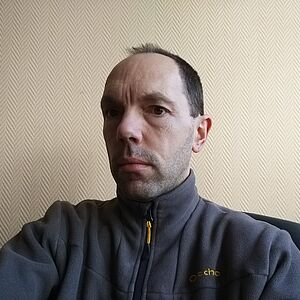## Paramétrage

### Accessibility# WilliamAlexandre

03.20.43.41.93
M2-314
Schools and divisions
Research topics Complex Analysis Keywords Complex analysis (Cauchy-Riemann's equations Regularity, problem of extension and of division in complex analysis, integral operators, residue currents).

## Working Areas

I work in complex analysis of several variables and in quasi complex geometry.  In complex analysis I use integral fomulae and residu currents which give explicit solutions of d-bar equation, of holomorphic extensions of functions defined on analytic varieties or division formulae. I established :

• optimal regularity of solutions of the Cauchy-Riemann equation d-bar u = f on a bounded domain D, of finite type, when the data f has certains type of regularity over D,
• necessary and sufficient or nearly sufficient conditions under which functions defined over the intersection of a domain D with a manifold ora singular varity has a holomorphic extension to D in L^p(D),
• caracterization of zero sets of H^p (D) functions when D is a bounded convex  domain of finite type,
• a proof of the H^p-corona theorem for 2 generators in convex domains of finite type,
• necessary and sufficient or nearly sufficient conditions for the existence of solutions to division problems of holomorphic functions in strictly pseudoconvex domains.

In quasi complex geometry, I prooved that there exsits hypersurfaces with constant rank which do not contain J-holomorphic disc with prescribed derivative at the origin. I also gave conditions under which such discs exist.